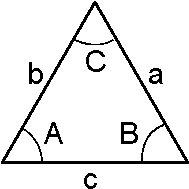# Law of Sines Formula

To calculate the unknown sides and angles of a triangle, the Law of Sines formula is used. The ratio of any side to the sine of the angle opposite to that side should be the same for all the sides of the triangle. If two sides are given and two angles of the triangle are given, then the unknown angle can be known using law of sines formula.The Law of Sines Formula is,

$\large \frac{a}{Sin\; A}=\frac{b}{Sin\; B}=\frac{c}{Sin\;C}$

### Solved Examples

Question 1: In a triangle ABC, the side lengths AB = 5 cm; BC = 3 cm and CA = 4 cm. The angle opposite to the side A is 60o. Calculate the other unknown angles?
Solution:

Given,
AB = a = 5 cm
BC = b = 3 cm
CA = c = 4 cm
A = 60o
The law of sines formula is,
$$\begin{array}{l}\frac{a}{sin A}\end{array}$$
=
$$\begin{array}{l}\frac{b}{sin B}\end{array}$$
=
$$\begin{array}{l}\frac{c}{sin C}\end{array}$$
$$\begin{array}{l}\frac{5 cm}{sin 60}\end{array}$$
=
$$\begin{array}{l}\frac{3 cm}{sin B}\end{array}$$
=
$$\begin{array}{l}\frac{4 cm}{sin C}\end{array}$$

$$\begin{array}{l}\frac{5 cm}{0.866}\end{array}$$
=
$$\begin{array}{l}\frac{3 cm}{sin B}\end{array}$$
=
$$\begin{array}{l}\frac{4 cm}{sin C}\end{array}$$

5.77 cm =
$$\begin{array}{l}\frac{3 cm}{sin B}\end{array}$$
=
$$\begin{array}{l}\frac{4 cm}{sin C}\end{array}$$
Solving 1st and 2nd term,

$$\begin{array}{l}\frac{3 cm}{sin B}\end{array}$$
= 5.77 cm

$$\begin{array}{l}\frac{3 cm}{5.77 cm}\end{array}$$
=
$$\begin{array}{l}sin B\end{array}$$
$$\begin{array}{l}sin B\end{array}$$
= 0.52
B = 31.33o

Solving 1st and 3rd term,

$$\begin{array}{l}\frac{4 cm}{sin C}\end{array}$$
= 5.77 cm

$$\begin{array}{l}\frac{4 cm}{5.77 cm}\end{array}$$
=
$$\begin{array}{l}sin C\end{array}$$
$$\begin{array}{l}sin C\end{array}$$
= 0.69
C = 43.63o